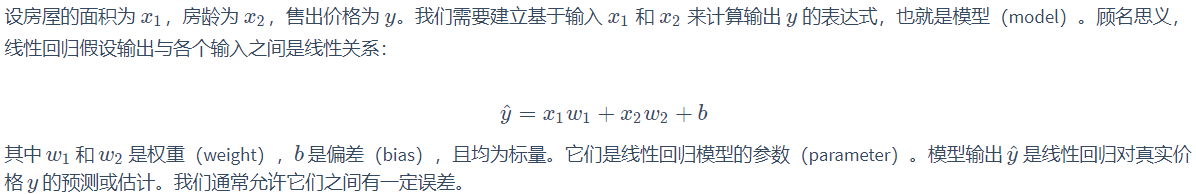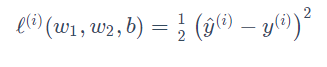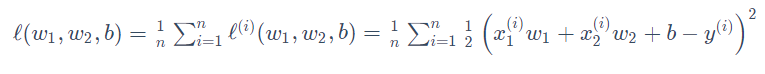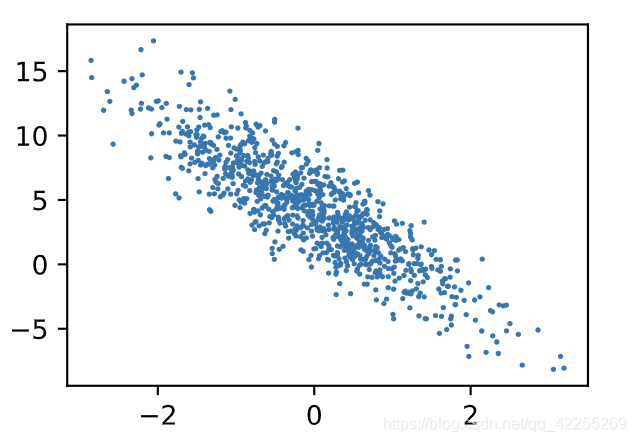# pytorch实现线性回归

## 1. 实现线性回归前的准备

``````import torch
from time import time

a = torch.ones(1000)
b = torch.ones(1000)
``````

``````start = time()
c = torch.zeros(1000)
for i in range(1000):
c[i] = a[i] + b[i]
print(time() - start)
``````

``````0.02039504051208496
``````

``````start = time()
d = a + b
print(time() - start) # 0.0008330345153808594
``````

## 定义一个房价预测问题，实例化应用场景：## 2. 线性回归的pytorch实现

``````%matplotlib inline
import torch
from IPython import display
from matplotlib import pyplot as plt # matplotlib包可用于作图，且设置成嵌入显示
import numpy as np
import random
``````

``````num_inputs = 2 #特征数量
num_examples = 1000 # 数据数量，即样本数量
true_w = [2, -3.4] # 线性回归模型真实权重
true_b = 4.2 # 偏差
features = torch.randn(num_examples, num_inputs,
dtype=torch.float32)
labels = true_w * features[:, 0] + true_w * features[:, 1] + true_b
# y=k*x+b
labels += torch.tensor(np.random.normal(0, 0.01, size=labels.size()),
dtype=torch.float32) # 噪声项，服从均值为0、标准差为0.01的正态分布。噪声代表了数据集中无意义的干扰
``````

features的每一行是一个长度为2的向量，而labels的每一行是一个长度为1的向量（标量）

``````def use_svg_display():
# 用矢量图显示
display.set_matplotlib_formats('svg') # 设置图类型为svg图

def set_figsize(figsize=(3.5, 2.5)):
use_svg_display()
# 设置图的尺寸
plt.rcParams['figure.figsize'] = figsize

set_figsize()
plt.scatter(features[:, 1].numpy(), labels.numpy(), 1) # 注意这里将tensor转化为numpy
````````````def data_iter(batch_size, features, labels):
num_examples = len(features)
indices = list(range(num_examples))
random.shuffle(indices)  # 样本的读取顺序是随机的
for i in range(0, num_examples, batch_size):
j = torch.LongTensor(indices[i: min(i + batch_size, num_examples)]) # 最后一次可能不足一个batch
yield  features.index_select(0, j), labels.index_select(0, j)
``````

``````batch_size = 10

for X, y in data_iter(batch_size, features, labels):
print(X, y)
break # 读取一个batch
``````

``````tensor([[-1.4239, -1.3788],
[ 0.0275,  1.3550],
[ 0.7616, -1.1384],
[ 0.2967, -0.1162],
[ 0.0822,  2.0826],
[-0.6343, -0.7222],
[ 0.4282,  0.0235],
[ 1.4056,  0.3506],
[-0.6496, -0.5202],
[-0.3969, -0.9951]])
tensor([ 6.0394, -0.3365,  9.5882,  5.1810, -2.7355,  5.3873,  4.9827,  5.7962,
4.6727,  6.7921])
``````

``````w = torch.tensor(np.random.normal(0, 0.01, (num_inputs, 1)), dtype=torch.float32)
b = torch.zeros(1, dtype=torch.float32)
``````

``````w.requires_grad_(requires_grad=True)
``````

``````def linreg(X, w, b):  # 矢量表达式

def squared_loss(y_hat, y):  # 损失函数
# 注意这里返回的是向量, 另外, pytorch里的MSELoss并没有除以 2
return (y_hat - y.view(y_hat.size())) ** 2 / 2

def sgd(params, lr, batch_size):  # 优化算法：小批量随机梯度下降算法
for param in params:
param.data -= lr * param.grad / batch_size # 注意这里更改param时用的是param.data

``````

``````lr = 0.03 # 学习率
num_epochs = 3
net = linreg
loss = squared_loss

for epoch in range(num_epochs):  # 训练模型一共需要num_epochs个迭代周期
# 在每一个迭代周期中，会使用训练数据集中所有样本一次（假设样本数能够被批量大小整除）。X
# 和y分别是小批量样本的特征和标签
for X, y in data_iter(batch_size, features, labels):
l = loss(net(X, w, b), y).sum()  # l是有关小批量X和y的损失
l.backward()  # 小批量的损失对模型参数求梯度
sgd([w, b], lr, batch_size)  # 使用小批量随机梯度下降迭代模型参数

# 不要忘了梯度清零
train_l = loss(net(features, w, b), labels)
print('epoch %d, loss %f' % (epoch + 1, train_l.mean().item()))
``````
``````epoch 1, loss 0.021578
epoch 2, loss 0.000096
epoch 3, loss 0.000050
``````

``````print(true_w, '\n', w)
print(true_b, '\n', b)
``````
``````[2, -3.4]
tensor([[ 1.9998],